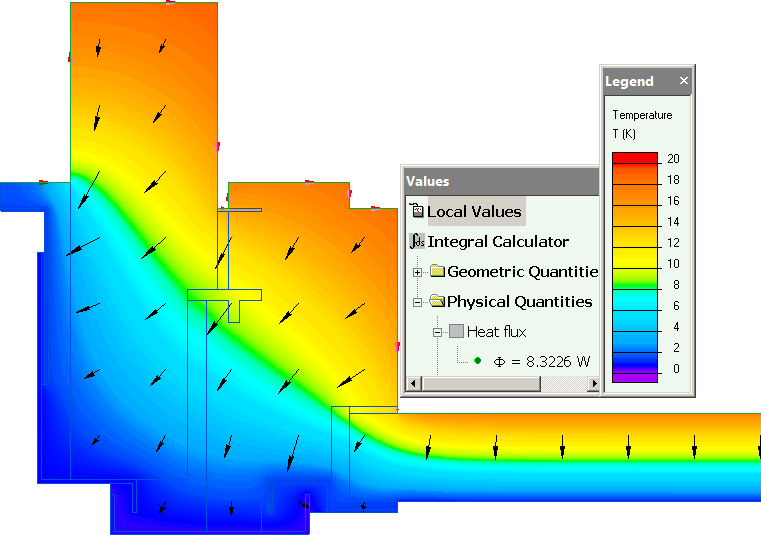QuickField

A new approach to field modelling

Main >> Applications >> Sample problems >> Thermal transmittance in frames. Test case D.5 validation

# ISO 10077-2:2012 Test case D.5 - Roof window frame section and insulation panel

QuickField simulation example

QuickField simulation of the test case "D.5. Roof window frame section and insulation panel" presented in ISO 10077-2:2012*

Problem Type
Plane-parallel problem of heat transfer.

Geometrytotal width b = 89 mm

Given
Thermal conductivity of EPDM, λ1 = 0.25 W/K-m.
Thermal conductivity of insulation panel, λ2 = 0.035 W/K-m.
Thermal conductivity of soft wood λ3 = 0.13 W/K-m.
Thermal conductivity of aluminium λ4 = 160 W/K-m.

Calculate two-dimensional thermal conductance L2D in accordance with ISO 10077-2:2012*.
(Reference thermal conductance L2D = 0.408 W/(m*K) )

Solution
Air contact surface thermal resistance Rs is caused by convention mechanism of heat transfer (contrary to conductivity mechanism in case of two solid bodies in contact).
The convection coefficient value is reciprocal to the surface resistance value:
α20 = 1/0.13 W/(K·m²),
α0 = 1/0.04 W/(K·m²).

Thermal conductivities of air gaps are adjusted to include the convection and radiation heat transfers in real situation, and correlated with the actual temperatures on the gap contours according to the recommendation of ISO 10077-2:2012. Equivalent air gap conductivity calculations are automated in the accompanying Excel spreadsheet.

Results
Heat flux and temperature distribution in frame section profile:Two-dimensional thermal conductance L2D = Heat flux per meter depth / Temperature difference = 8.32 / 20 = 0.416 W/(m*K).
Difference between the calculated and reference values is less than 3%. This simulation accuracy complies with the requirements of ISO 10077-2:2012.

* ISO 10077-2:2012 Thermal performance of windows, doors and shutters -- Calculation of thermal transmittance -- Part 2: Numerical method for frames.